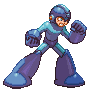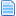AI Detect Enemy's (sctrl) Projectile System (Read 1657 times)

Started by ink, October 02, 2018, 05:02:54 pm

inkAI Detect Enemy's (sctrl) Projectile System
#1  October 02, 2018, 05:02:54 pm
This is a helper that stays bound to the character to detect if an enemy spawns a (sctrl) Projectile. Once the character is hit once by the enemy's projectile the data recorded can be used to find out a lot more about the projectile. Including velocity, distance, position on screen etc.

This system does NOT use any root variable but does need one blank anim.

There are plenty of notes included. If anyone thinks of a way to improve this let me know!
Also check out my AI Detect Enemy's (Helper) Projectile System

This goes in statedef -3
Code:
`[state -2, AI Detect Projectile System]type = helpertrigger1 = ailevel && !numhelper(33333332)name = "AI Detect Projectile System"ID = 33333332stateno = 75475890postype = p1ownpal = 1keyctrl = 0size.xscale = 1.0size.yscale = 1.0supermovetime = 2147483647pausemovetime = 2147483647ignorehitpause = 1`

This goes in CNS
Code:
`;==========================================================================;Inktrebuchet ;Learning AI 10/10/2018                  ;==========================================================================;;==========================================================================;Goal:;-Detect enemy's projectile (sctrl). For use with AI. 敵の発射物（sctrl）を検出する。 AIで使用する場合;;==========================================================================;notes:;enemy projectile data can not be saved from round to round... without using root variables;These calculations are based on the projectile needing to hit you. ;This is meant to detect one projectile at a time.;Projectiles that stay in place may throw off projdist x.;This does not account for proj(sctrl)s that are not used for projectiles.;This is compatibility with Inktrebuchet's "AI Detect Projectile (helper) System";;Base examples of trigger use: ;;Enemy's projectile helper's distance from character.;trigger1 = enemy,NumProj = 1;trigger1 = helper(33333332),fvar(9) ;(enemy,projdist x);;;==========================================================================;                Detect Enemy's Projectile Sctrl var usage.;==========================================================================; numhelper(33333332) Variable usage:; This is a record of the variables that are used;;var(0) - sets/changes var number;var(1) - used in statedef 75475892 to help save (enemy,projpos y) to correct slot.;var(2) - enemy facing when projectile is spawned.;var(3) - var(9) -;var(10) - var(19) - amount of time projectile existed.;var(50) - var(59) - enemy's projectile state;;fvar(0) - fvar(6)  -;fvar(7) - best guess of enemy projectiles pos y.(enemy,projpos y) *projbodydist y;fvar(8) - enemy projectile's velocity (enemy,projvel x);fvar(9) - enemy projectiles distance from you. (enemy,projdist x);fvar(10) - fvar(19) - enemy's distance at start of projectile slots;fvar(20) - fvar(29) - best guess of enemy projectiles pos y, based on you being hit by enemy's projectile. (enemy,projpos y) slots.; ;-------------------------------------------------------------------------- [statedef 75475890]anim = 9741 ;blank[state 75475890, clip board] type = DisplayToClipboardtrigger1 = 1text = "enemy,projvel x = ???????, enemy,projdist x = ???????\nenemy,projpos y = ???????";params = [State 75475890, BindToRoot]type = BindToRoottrigger1 = 1time = 1facing = 1pos =0,-5ignorehitpause =1 persistent =1 [state 75475890, 0] ; sets var numbertype = nulltrigger1 = var(0) = 0 trigger1 = var(0) := 29[state 75475890, 1] ; This changes which slot the sprojectiles info is recorded in. type = nulltriggerall = time = 0trigger1 = var(0) = 30 ;this is need for statedef 75475891, if enemy's projectile doesn't make contact and var(0) is at 59 + 1.trigger1 = var(0) := 29trigger2 = var(var(0)+30) > 0trigger2 = prevstateno = 75475891 || prevstateno = 75475898trigger2 = var(0) > 20 ; prevent var(0) from going past 50trigger2 = var(0) := var(0) - 1trigger3 = fvar(8) := 0 ;reset trigger4 = fvar(9) := 0 ;reset [state 75475890, 2] ; reset, this is need for statedef 75475891, if enemy's projectile doesn't make contact.type = nulltriggerall = time = 1 ;<<<<<<<<<<<<<<<<<<<<<<<<<<<<<<<<<<<<<<<<<<<<<<<<<<<<<<<<<<<<<<<<<<<<<< could be 0 ????????trigger1 = var(var(0)+30) := 0 ;reset, enemy projectile didn't hit youtrigger2 = var(var(0)-10) := 0 ;reset, enemy projectile didn't hit you[state 75475890, 3] ;enemy projectile exists and HAS been recorded.type = changestate;A projectile that has been recorded is being used by the enemytrigger1 = enemy,NumProj = 1trigger1 = enemy,stateno != 0 ; This is to prevent a trigger from an empty var = enemy,stateno.trigger1 = var(59) = enemy,stateno || var(58) = enemy,stateno || var(57) = enemy,stateno || var(56) = enemy,stateno || var(55) = enemy,stateno || var(54) = enemy,stateno || var(53) = enemy,stateno || var(52) = enemy,stateno || var(51) = enemy,stateno || var(50) = enemy,statenovalue = 75475892[state 75475890, 4] ;enemy projectile exists and HAS NOT been recorded.type = changestate;Enemy has used a projectile that's not recorded.trigger1 = enemy,NumProj = 1trigger1 = enemy,stateno != 0 ; This is to prevent a trigger from an empty var = enemy,stateno.trigger1 = var(59) != enemy,stateno || var(58) != enemy,stateno || var(57) != enemy,stateno || var(56) != enemy,stateno || var(55) != enemy,stateno || var(54) != enemy,stateno || var(53) != enemy,stateno || var(52) != enemy,stateno || var(51) != enemy,stateno || var(50) != enemy,statenovalue = 75475891[state compatibility] ;compatibility with Inktrebuchet's "AI Detect Projectile System". System must be installed to work.type = changestatetrigger1 = root,numhelper(33333333) = 1 ; "AI Detect Projectile System" existstrigger1 = PlayerIDExist(helper(33333333),var(3)) ;enemy's helper projectile exists.value = 75475899;--------------------------------------[statedef 75475899] ;compatibility with Inktrebuchet's "AI Detect Projectile System".[state compatibility] ;compatibility with Inktrebuchet's "AI Detect Projectile System". System must be installed to work.type = nulltrigger1 = root,numhelper(33333333) = 1 ; "AI Detect Projectile System" existstrigger1 = PlayerIDExist(helper(33333333),var(3)) ;enemy's helper projectile exists.trigger1 = fvar(8) := PlayerID(helper(33333333),var(3)),vel xtrigger1 = fvar(9) := PlayerID(helper(33333333),var(3)),p2bodydist x ;enemy,pos xtrigger1 = fvar(7) := PlayerID(helper(33333333),var(3)),pos y[state 75475892, clip board] type = DisplayToClipboardtrigger1 = 1text = "enemy,projvel x = %d, enemy,projdist x = %d\nenemy,projpos y = %d"params = fvar(8), fvar(9) , fvar(7)[state compatibility] ;compatibility with Inktrebuchet's "AI Detect Projectile System". System must be installed to work.type = changestatetrigger1 = PlayerIDExist(helper(33333333),var(3)) = 0 ;enemy's helper projectile exists.value = 75475890;--------------------------------------[statedef 75475892] ; A projectile that has been recorded is being used by the enemy[state 75475892, clip board] type = DisplayToClipboardtrigger1 = 1text = "enemy,projvel x = %d, enemy,projdist x = %d\nenemy,projpos y = %d"params = fvar(8), fvar(9) , fvar(7)[State 75475892, BindToEnemy]type = BindToRoottrigger1 = time = 0time = 1facing = -1pos =p2bodydist x,0ignorehitpause =1 persistent =1 [state 75475892, 0] ; enemy facing when projectile is spawned.type = nulltriggerall = time = 0trigger1 = var(2) := cond(enemy,facing = root,facing, -1, 1) [state 75475892, 1] ; caluculates enemy,projectile's velocity.type = nulltriggerall = time = 0trigger1 = enemy, stateno = var(50) trigger1 = fvar(8) := fvar(10) / var(10) ; velocity of projectiletrigger1 = var(1) := 50 ;used to find enemy,projpos ytrigger2 = enemy, stateno = var(51)trigger2 = fvar(8) := fvar(11) / var(11) ; velocity of projectiletrigger2 = var(1) := 51 ;used to find enemy,projpos ytrigger3 = enemy, stateno = var(52)trigger3 = fvar(8) := fvar(12) / var(12) ; velocity of projectiletrigger3 = var(1) := 52 ;used to find enemy,projpos ytrigger4 = enemy, stateno = var(53)trigger4 = fvar(8) := fvar(13) / var(13) ; velocity of projectiletrigger4 = var(1) := 53 ;used to find enemy,projpos ytrigger5 = enemy, stateno = var(54)trigger5 = fvar(8) := fvar(14) / var(14) ; velocity of projectiletrigger5 = var(1) := 54 ;used to find enemy,projpos ytrigger6 = enemy, stateno = var(55)trigger6 = fvar(8) := fvar(15) / var(15) ; velocity of projectiletrigger6 = var(1) := 55 ;used to find enemy,projpos ytrigger7 = enemy, stateno = var(56)trigger7 = fvar(8) := fvar(16) / var(16) ; velocity of projectiletrigger7 = var(1) := 56 ;used to find enemy,projpos ytrigger8 = enemy, stateno = var(57)trigger8 = fvar(8) := fvar(17) / var(17) ; velocity of projectiletrigger8 = var(1) := 57 ;used to find enemy,projpos ytrigger9 = enemy, stateno = var(58)trigger9 = fvar(8) := fvar(18) / var(18) ; velocity of projectiletrigger9 = var(1) := 58 ;used to find enemy,projpos ytrigger10 = enemy, stateno = var(59)trigger10 = fvar(8) := fvar(19) / var(19) ; velocity of projectiletrigger10 = var(1) := 59 ;used to find enemy,projpos y[state 75475892, 3] ; calculates enemy,projectile's distance. type = nulltrigger1 =  time = 1 ; has to be 1 because bindtoroot is slow.trigger1 =  fvar(9) := rootdist x - fvar(8); subtract fvar(8) to correct 1 tick delay above.trigger2 = fvar(9) := fvar(9) - fvar(8) * var(2)[state 75475892, 4] ; you got hit by enemy's projectile and possibly captured its pos y. type = nulltriggerall = enemy,projcontact = 1 <= 1 trigger1 = fvar(var(1)-30) > root,pos y  trigger1 = fvar(var(1)-30) := root,pos ytrigger1 = fvar(7) := fvar(var(1)-30)ignorehitpause =1 [state 75475892, 5]type = changestatetrigger1 = enemy,NumProj = 0value = 75475890;--------------------------------------[statedef 75475891] ; Enemy has used a projectile that's not recorded. Record enemy's projectile, the distance it was created at and how long in existed.[state 75475891, clip board] type = DisplayToClipboardtrigger1 = 1text = "enemy,projvel x = ......., enemy,projdist x = .......\nenemy,projpos y = .......";params = [State 75475891, BindToEnemy] type = BindToRoottrigger1 = time = 0time = 1facing = -1pos =p2bodydist x,0ignorehitpause =1 persistent =1 [state 75475891, 0] ; record enemy's statenotype = nulltrigger1 = time = 0 trigger1 = var(var(0)+30) := enemy,stateno [state 75475891, 1] type = nulltrigger1= enemy,NumProj = 1;records enemy's projectile state and time that projectile existed. slots: var(50) through var(59)trigger1 = var(var(0)-10) := var(var(0)-10) + 1 ;amount of time projectile exsited for.trigger1 = fvar(var(0)-10) := rootdist x ;this is used as distance. [state 75475891, 2] type = changestate;enemy's projectile made contact with youtrigger1 = root,movetype = H ; trigger1 = enemy,projcontact = 1 <= 1 trigger1 = fvar(var(0)) := cond(root,pos y = 0, -1, root,pos y) ; best guess of enemy projectiles pos y.(enemy,projpos y)trigger1 = fvar(7) := fvar(var(0)) ; best guess of enemy projectiles pos y.(enemy,projpos y)value = 75475890[state 75475891, 3] type = changestate;enemy's projectile did NOT make contact.trigger1 = enemy,NumProj = 0value = 75475898;--------------------------------------[statedef 75475898] [state 75475898] type = changestate;enemy's projectile did NOT make contact.trigger1 = var(0) := var(0) + 1 ;resetvalue = 75475890`

Example of Use:
Code:
`[state -1] ;Jump over enemy projectilestype = changestatetriggerall = AILeveltriggerall = statetype = Striggerall = ctrltrigger1 = numhelper(33333332) = 1 ;Projectile Detect existstrigger1 = helper(33333332), fvar(9) > 0 ; enemy's projectile exists. *helper(33333332), fvar(9) = (enemy,projdist x)trigger1 = helper(33333332),fvar(8) > 0 ; enemy's projectile vel x is greater than 0;enemy,projdist x / enemy,projvel x = max jump height / abs(const(velocity.jump.y)).trigger1 = helper(33333332),fvar(9) / helper(33333332),fvar(8) = 100 / abs(const(velocity.jump.y)) ; 100=max jump heightvalue = 40`
Last Edit: December 11, 2018, 07:14:52 am by ink

DarkWolf13Re: AI Detect Enemy's (sctrl) Projectile System
#2  May 19, 2019, 02:56:10 am
Thanks to your help with an edited code, this should help people with their characters with projectile deflecting moves
Code:
`[state -1] ;Counter sctrl proj last second. type = changestatetriggerall = AILeveltriggerall = statetype !=Atriggerall = ctrltrigger1 = numhelper(33333332) = 1 ;Projectile Detect existstrigger1 = helper(33333332), fvar(9) > 0 ; enemy's projectile exists. *helper(33333332), fvar(9) = (enemy,projdist x)trigger1 = helper(33333332),fvar(8) > 0 ; enemy's projectile vel x is greater than 0;enemy,projdist x / enemy,projvel x = active move time.trigger1 = helper(33333332),fvar(9) / helper(33333332),fvar(8) = [2,15] ; 2,15 is the reflector’s active frames. Note by DarkWolf13: Increase the last number to make them respond sooner.value = 1500`
This was from my edited version of Chotto-Komaru's Fox for my VSD Tag Project. Deep down... thank you. This is really gonna make the AI for the projectile-deflecting fighters in my project more challenging.
Beware the Dark Wolf once more!